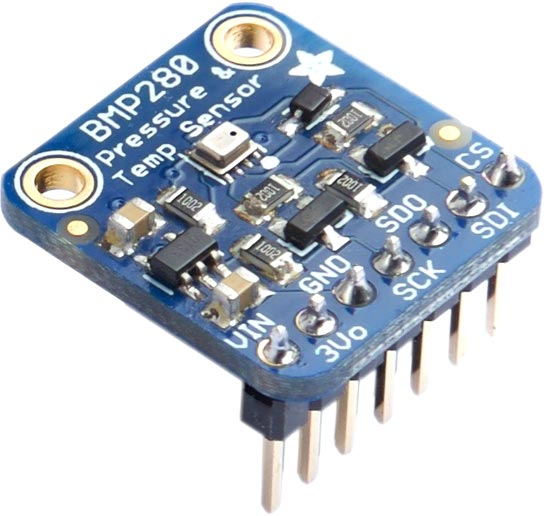## BMP280 Pressure Sensor

The Bosch BMP280  can measure barometric pressure with ±1 hPa absolute accuracy, and temperature with ±1.0 °C accuracy. You can also use it as an altimeter with  ±1 metre accuracy. It supports both I2C and SPI interfaces.

Breakout boards are available from Adafruit  and other suppliers:The I2C address is #x77 or 119.

This sensor requires floating-point calculations, so it's only suitable for 32-bit versions of uLisp. The routines implement the calculations given in the datasheet, but using uLisp's single-precision floating point.

#### Routines

First the following useful routines are defined for reading unsigned and signed integers:

```(defun uint16 (str) (logior (read-byte str) (ash (read-byte str) 8)))

(defun int16 (str) (logior (read-byte str) (ash (ash (read-byte str) 24) -16)))

(defun uint20 (str)
(logior
(logand (ash (read-byte str) -4) #x0F)))```

#### Initialising the sensor

The routine bmp280-setup resets the sensor, and then reads the temperature and pressure configuration values from the PROM into the lists tcomp and pcomp. You should call this once before using the sensor:

```(defvar tcomp nil)

(defvar pcomp nil)

(defun bmp280-setup ()
(with-i2c (str 119)
; Set no oversampling, normal mode
(write-byte #xF4 str)
(write-byte #b00100111 str)
(restart-i2c str)
(write-byte #x88 str)
(restart-i2c str 24)
(setq tcomp (list (uint16 str) (int16 str) (int16 str)))
(setq
pcomp
(list
(uint16 str) (int16 str) (int16 str) (int16 str) (int16 str)
(int16 str) (int16 str) (int16 str) (int16 str)))))```

For example:

```> (bmp280-setup)
(37577 -10537 3024 6631 -64 -7 15500 -14600 6000)```

The following routine bmp280-t returns the temperature in °C. This must be called before reading the pressure to set the variable temp-fine:

```(defvar temp-fine 0)

(defun bmp280-t ()
(let ((tn (lambda (n) (nth (1- n) tcomp))))
(delay 2)
(with-i2c (str 119)
(write-byte #xfa str)
(restart-i2c str 3)
(let* ((temp (uint20 str))
(v1 (* (- (/ temp 16384) (/ (tn 1) 1024)) (tn 2)))
(v1a (- (/ temp 131072) (/ (tn 1) 8192)))
(v2 (* v1a v1a (tn 3)))
(tf (+ v1 v2)))
(setq temp-fine tf)
(/ tf 5120)))))```

For example:

```> (bmp280-t)
24.5578```

It's 24.5°C.

The following routine bmp280-p returns the pressure in hPa:

```(defun bmp280-p ()
(let ((pn (lambda (n) (nth (1- n) pcomp))))
(with-i2c (str 119)
(write-byte #xf7 str)
(restart-i2c str 3)
(let* ((p (uint20 str))
(v1 (- (/ temp-fine 2) 64000))
(v2 (/ (* v1 v1 (pn 6)) 32768)))
(setq v2 (+ v2 (* v1 (pn 5) 2)))
(setq v2 (+ (/ v2 4) (* (pn 4) 65536)))
(setq v1 (/ (+ (/ (* (pn 3) v1 v1) 524288) (* (pn 2) v1)) 524288))
(setq v1 (* (+ 1 (/ v1 32768)) (pn 1)))
(when (zerop v1) (print "error"))
(setq p (- 1048576 p))
(setq p (/ (* (- p (/ v2 4096)) 6250) v1))
(setq v1 (/ (* (pn 9) p p) 2147483648))
(setq v2 (/ (* p (pn 8)) 32768))
(/ (+ p (/ (+ v1 v2 (pn 7)) 16)) 100)))))```

For example:

```> (bmp280-p)
1012.3```

1. ^ BMP280 Datasheet on Adafruit.
2. ^ Adafruit BMP280 I2C or SPI Barometric Pressure & Altitude Sensor on Adafruit.